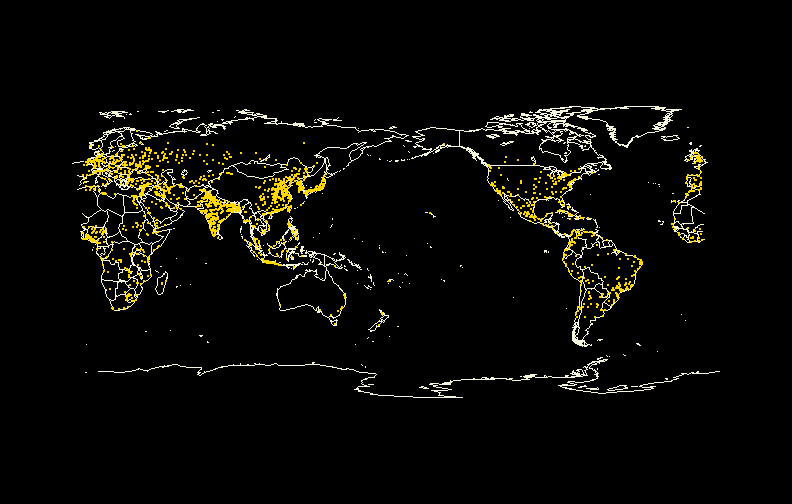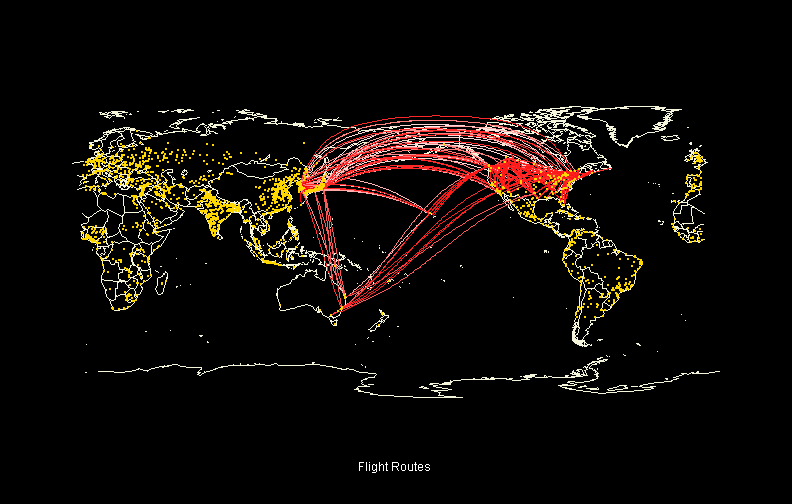## Saturday, 4 January 2014

### Drawing Great Circle in R - Flight Map

'Great Circle' is usually drawn on a map of world to display flight paths between cities. This is useful when there are multiple flights between same cities or in the nearby cities where the straight lines often overlap and do not display the fact that there are more than one flights represented.

Firstly, the packages I used include:

• 'maps'
• 'plyr'
• 'sp'
• 'geosphere'
The data I have used in the below examples came from http://openflights.org/data.html which provided information about airlines, airports and flight routes in separate files. The data is structured in a way that makes merging fairly easy.

airline <- read.csv("Data/Airlines.csv", sep = ",", header = TRUE) airport <- read.csv("Data/Airport.csv", sep = ",", header = TRUE) route <- read.csv("Data/Routes.csv", sep = ",", header = TRUE) A <- airport[, c("ID", "Airport", "City", "Country", "Lat", "Lon")] colnames(A) <- paste("Dep", colnames(A), sep = "_") Dat <- merge(route[, c("Airline_ID", "Dep_Airport_ID", "Arr_Airport_ID")], A, by.x = "Dep_Airport_ID", by.y = "Dep_ID") A <- airport[, c("ID", "Airport", "City", "Country", "Lat", "Lon")] colnames(A) <- paste("Arr", colnames(A), sep = "_") Dat <- merge(Dat, A, by.x = "Arr_Airport_ID", by.y = "Arr_ID") Dat <- merge(Dat, airline[, c("ID", "Airline", "Active")], by.x = "Airline_ID", by.y = "ID")

As it would make little sense to draw all flight paths around the world, I have filtered the data to selected countries to be used in the example.

DatX <- Dat[which(Dat\$Dep_Country != Dat\$Arr_Country), ] DatX <- DatX[which(DatX\$Dep_Country %in% c("Canada", "Australia", "South Korea", "United States", "Japan") & DatX\$Arr_Country %in% c("Canada", "Australia", "South Korea", "United States", "Japan")), ]

To distinguish between flight paths, I have used the colour degradation between red and white, as there are too many flight paths to colour code them with different colours.

col_gradient <- colorRampPalette(c("white", "red")) Colours <- col_gradient(length(unique(DatX\$Airline_ID))) DatX\$Colour <- Colours[as.numeric(factor(as.character(DatX\$Airline_ID)))]

The below code calculates the projection of great circles to correspond to flight routes. In essence, I have assigned 100 points between the destination cities to connect in order to form a great circle, and repeated this for all flight destinations in the dataset. The tricky part is that depending on the centre of the world map (e.g. Pacific-centred or Atlantic-centred), you may need to recalculate the longitude as the map does not show negative longitudes and may result in disconnected or distorted great circles .

GC_Dat <- list()

for (j in 1:nrow(DatX)) {
X <- as.data.frame(gcIntermediate(DatX[j, c("Arr_Lon", "Arr_Lat")], DatX[j,
c("Dep_Lon", "Dep_Lat")], n = 100, addStartEnd = TRUE))

R <- nrow(X[which(X\$lon < 0), ])
if (R == 0) {
RR <- X
}
if (R > 0) {
a <- ifelse(X\$lon >= 0, X\$lon, X\$lon + 360)

DF <- diff(a)
L <- length(DF) - length(DF[which(DF < 0)])

if (L == 0 | L == length(DF)) {
X\$lon <- a
RR <- X
}
if (L != 0 & L != length(DF)) {
aa <- X[which(X\$lon >= 0), ]
b <- X[which(X\$lon < 0), ]
if (nrow(b) > 0) {
B <- b[nrow(b), ]

b\$lon <- b\$lon + 360
B\$lon <- 360

b <- rbind(b, B)
b\$id <- b\$id + 0.5
}

RR <- rbind(aa, b)
}
}

RR\$Airline_ID <- DatX[j, "Airline_ID"]

GC_Dat[[j]] <- merge(DatX[j, ], RR, by = "Airline_ID")
}

Now that the data preparation is completed, we need to plot the world map as a below.

map("world2", col = "beige", bg = "black")

To make it look pretty, the following codes will show top 5% cities by population on the map.

data(world.cities) Cities <- world.cities Maj_Cities <- Cities[which(Cities\$pop >= quantile(Cities\$pop, prob = 0.95)), ] Maj_Cities\$long <- ifelse(Maj_Cities\$long >= 0, Maj_Cities\$long, Maj_Cities\$long + 360) map("world2", col = "beige", bg = "black") points(Maj_Cities\$long, Maj_Cities\$lat, pch = 16, cex = 0.3, col = "gold")Finally, the flight routes as represented by the great circles are laid on top of the map.

map("world2", col = "beige", bg = "black") for (i in 1:length(GC_Dat)) { lines(lat ~ lon, GC_Dat[[i]], lty = 1, col = unique(GC_Dat[[i]]\$Colour)) } points(Maj_Cities\$long, Maj_Cities\$lat, pch = 16, cex = 0.3, col = "gold") title(sub = "Flight Routes", col.sub = "white")